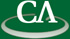## Multicore Algorithm Design

#### Roman Pearce, CECM, Simon Fraser.

Wednesday February 10th, in K9509 at 3:30pm.

```Abstract.
In this talk we will describe the principles at work in the
design and implementation of high performance, fine grained
parallel programs for multicore processors.  In addition to
example code that you can try yourself, we will discuss two
real problems where these techniques were successfully used.

Given two sparse polynomials f = f_1 + f_2 + ... + f_n and
g = g_1 + g_2 + ... + g_m, our first problem is to compute
the product f x g.  We compute, sort, and merge all pairwise
products f_i x g_j in parallel while writing out the result.
Our next problem is to divide f/g and compute the quotient
q with f - q g = 0.  The quotient is computed term by term
while it is being multiplied by g and subtracted from f in
parallel, with no redundant work.  Both algorithms achieve
superlinear speedup on an Intel Core i7 CPU with 4 cores.

```